# 一. 神经网络

## （一）基础知识

### 2. 神经网络的基本介绍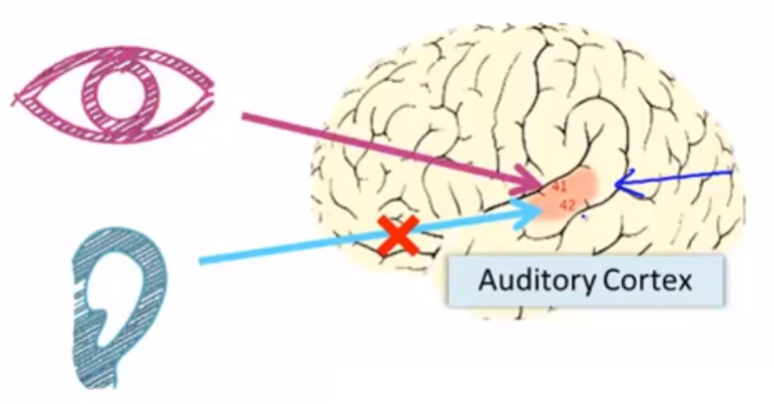### 3.利用神经网络的小发明### 4. 一些基础概念

1）ReLU： Rectified Linear Unit ——修正线性单元

2） Drop-out正则化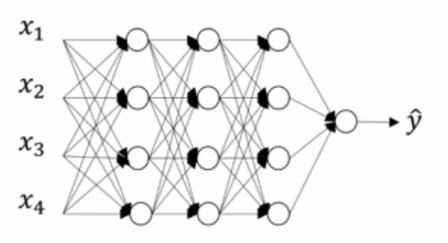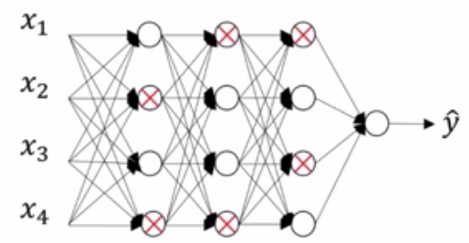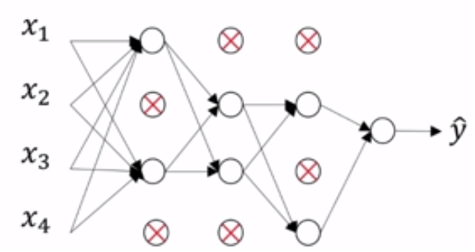3） Inverted DropOut： 反向随机失活
3 = np.randm.rand(a3.shape, a3.shape) < keep_prob
a3 = np.multiply(a3, d3)
a3 /= keep-prob

4） 梯度下降的优化算法——指数加权平均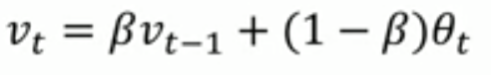θ_t：为第 t 天的实际观察值，
V_t: 是要代替 θ_t 的估计值，也就是第 t 天的指数加权平均值，
β： 为 V_{t-1} 的权重，是可调节的超参。( 0 < β < 1 )

eg：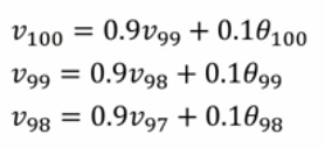5）梯度下降优化算法 —— 动量梯度算法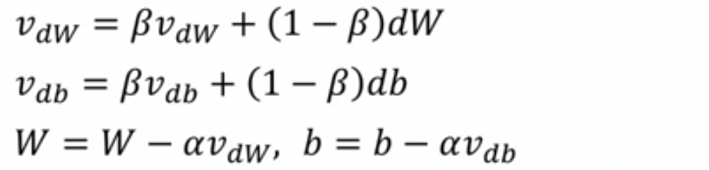6） RMSprop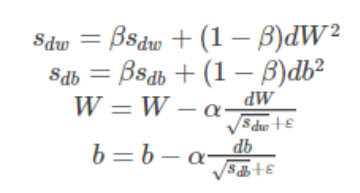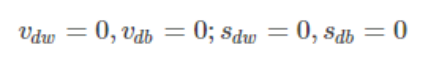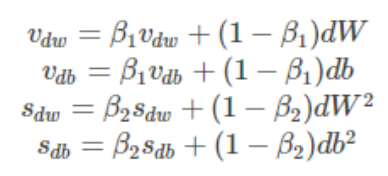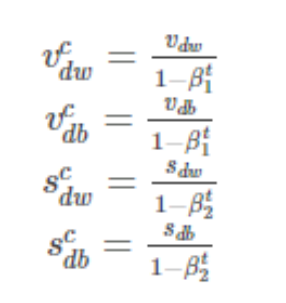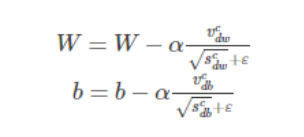8） softmax函数
softmax函数是对向量进行归一化处理，凸显其中最大的值并抑制远低于最大值的其他分量。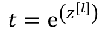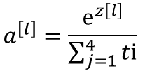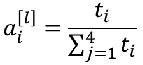eg：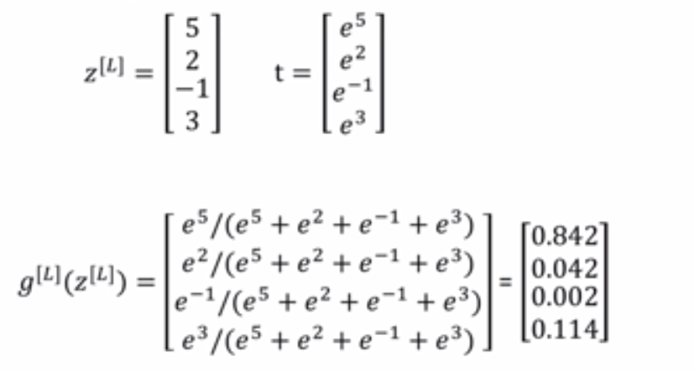## （二）神经网络的计算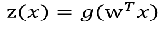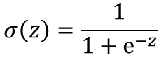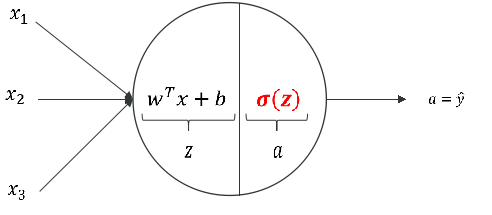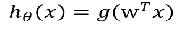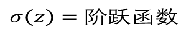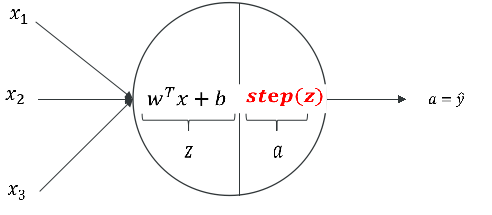ps： 常用的阶跃函数：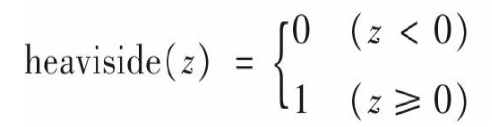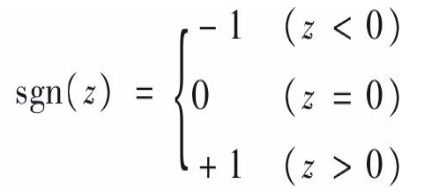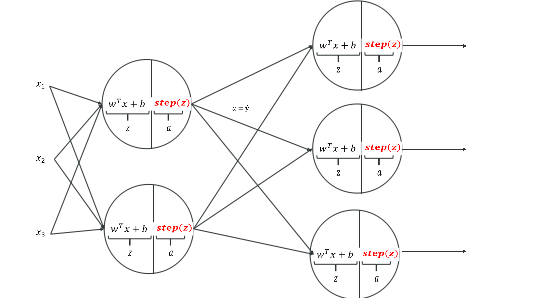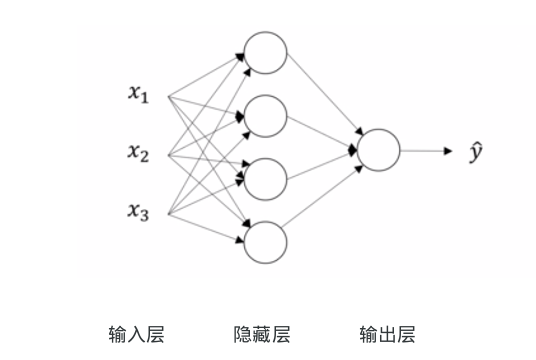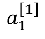表示第一层中的第一个神经元

### 一个神经元的表达式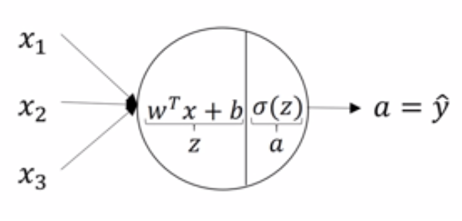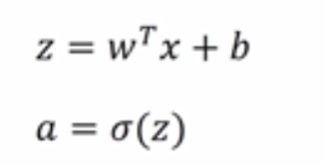计算顺序： 输入计算第一层第一个，计算完第一个再计算第二个，依次类推，层数也一样，计算完第一层再计算第二层直到输出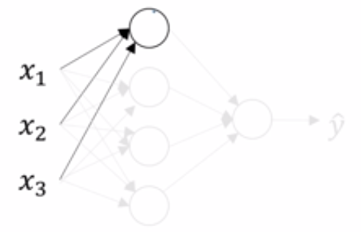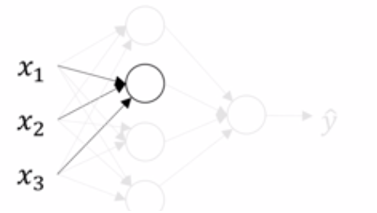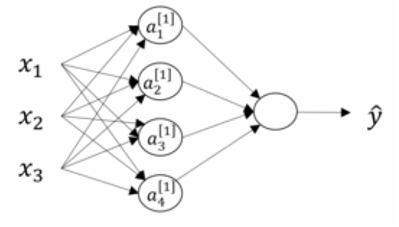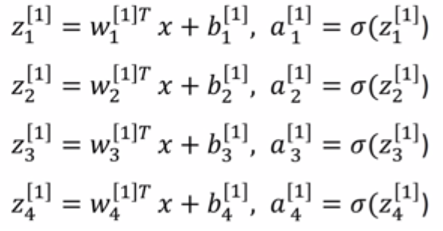### 神经网络的表示

eg：ps： W矩阵的形状：（本层神经元的个数，上一层的输入值数量）

### 激活函数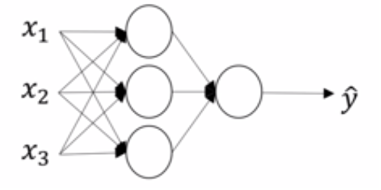1. 非线性变化
2. 梯度下降
在神经网络发展过程中将激活函数从阶跃函数改成逻辑函数是很大的进步，目的是为了实现梯度下降

1）Heaviside（Step）阶跃函数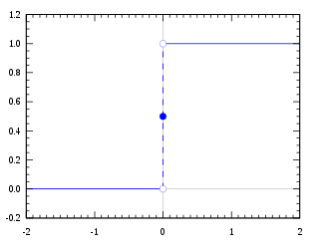2）SIgmod函数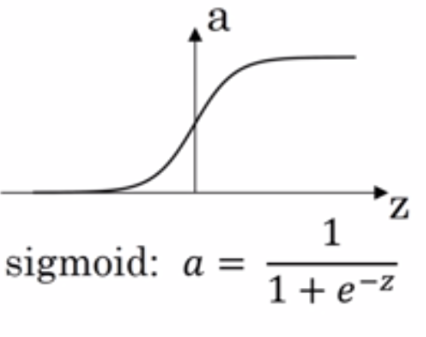3）tanh函数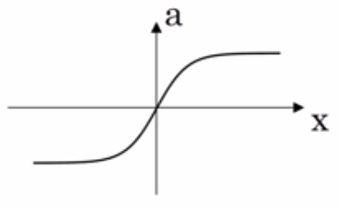4）ReLu函数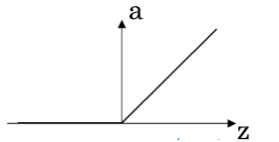5）Leaky ReLu 函数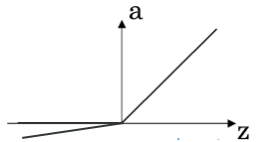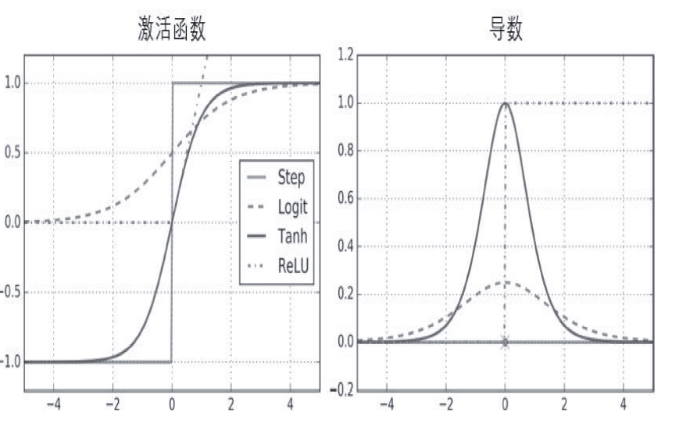1. Step函数的导数一直为0
2. Tanh输出值是（0,1）
3. Relu没有最大输出值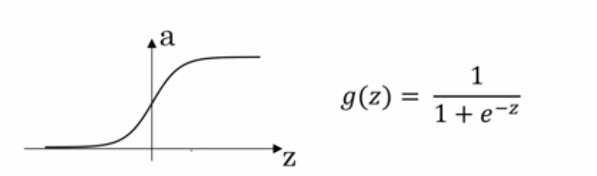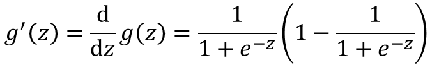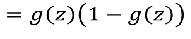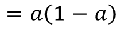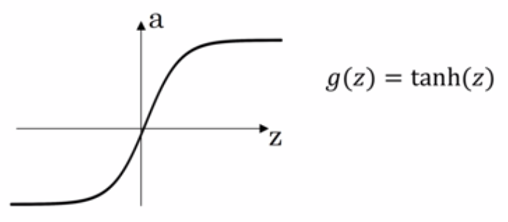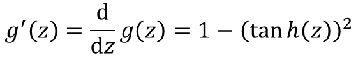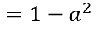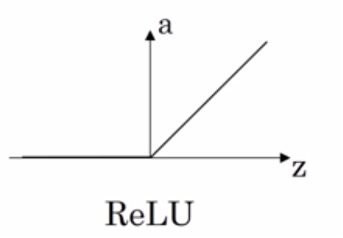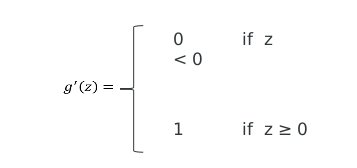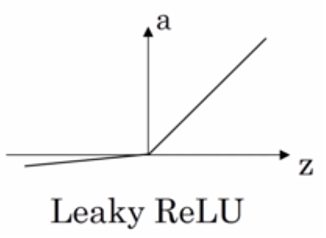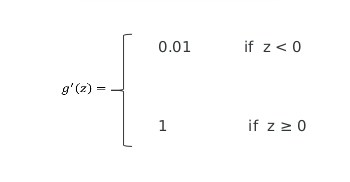### 神经网络传播方向

#### 1） 正向传播

（1） 对神经网络第一层计算
1-1 对第一层的第一个进行计算

• 应用线性函数计算
• 应用非线性函数进行计算（激活函数： sigmod，Tanh，ReLu，Leaky ReLu）

1-2 对第一层的第二个进行计算
1-3 对第一层的第三个进行计算

（2）对神经网络第二层进行计算

（3）对神经网络第三层进行计算

#### 2）反向传播

##### 计算图

eg：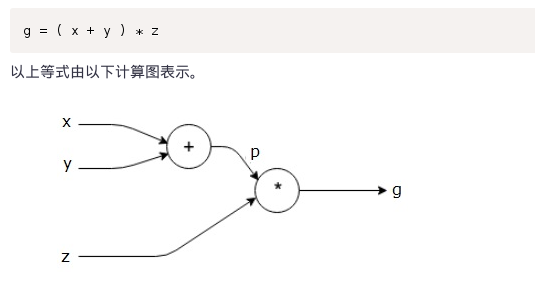eg：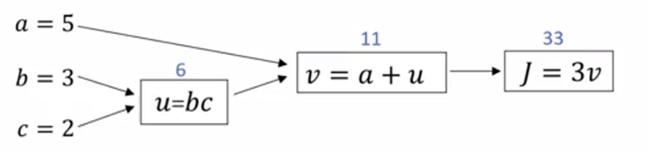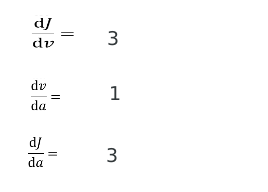##### 神经网络的梯度下降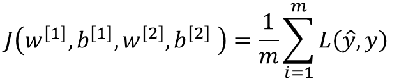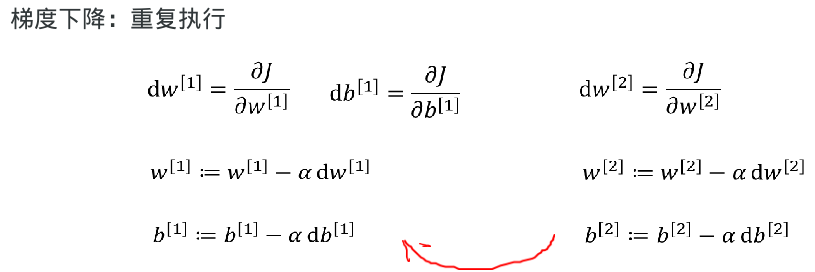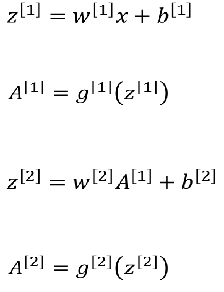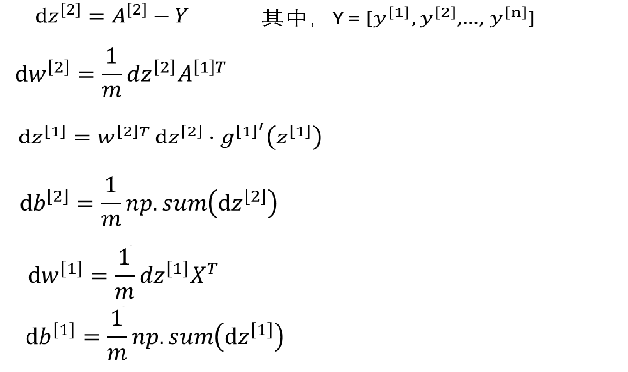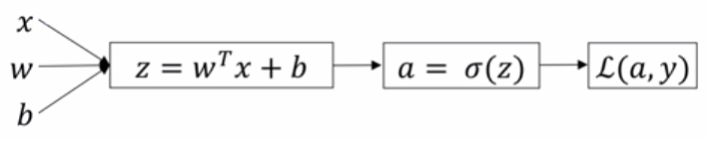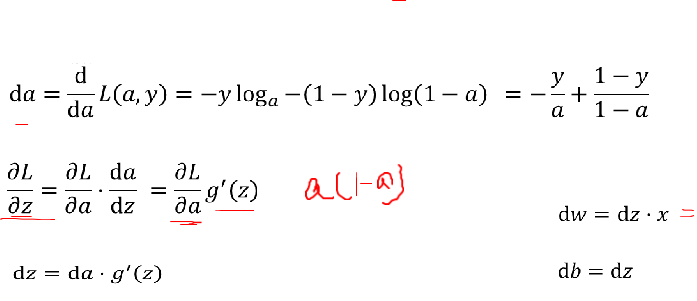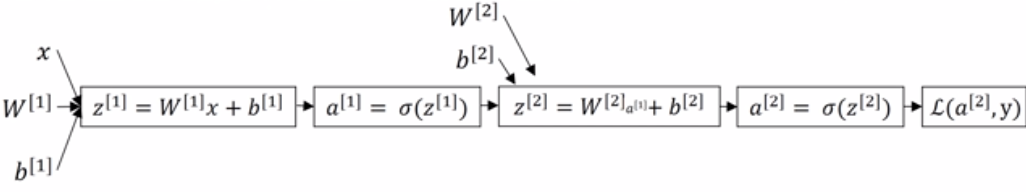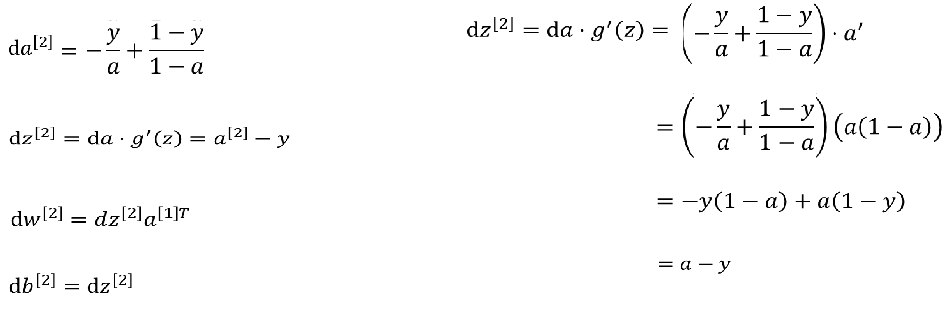（1）对最后一层进行求导
1-1 我们已知的，不用求损失函数；我们将损失函数求导
1-2 dL / da , a = SIngmod(z)
1-3 dL / dz = ( dL / da ) * (da / dz)
1-4 dL / dw = ( dL / da ) * (da /dz) * (dz/dw)
dL / db = ( dL /da ) * ( da / dz ) * (dz / db)
（2）对倒数第二层进行求导
1-1 dL / w = dL / dz * dz  / da * da[1 ]/ dz * dz / dw
1-2 dL / b = dL / dz * dz / da * da / dz * dz / db

# 二. tensorflow 模块

Tensorflow是一种计算图模型，即用图的形式来表示运算过程的一种模型。Tensorflow程序一般分为图的构建和图的执行两个阶段。图的构建阶段也称为图的定义阶段，该过程会在图模型中定义所需的运算，每次运算的的结果以及原始的输入数据都可称为一个节点（operation ，缩写为op）。

## （1） 查看tensorflow的版本

import tensorflow
print(tensorflow.__version__)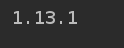## （2）简单应用tensorflow

variable： 是一个变量

import tensorflow as tf

with tf.device('/cpu:0'):
x = tf.Variable(3, name='x')
y = tf.Variable(4, name='y')
f = x * x * y + y + 2

# 配置一个计算图的上下文环境
# 配置里面是具体运行过程在那里执行给打印出来
sess = tf.Session(config=tf.ConfigProto(log_device_placement=True))
# 碰到sess.run()就会立刻去调用计算
sess.run(x.initializer)
sess.run(y.initializer)
result = sess.run(f)
print(result)
sess.close()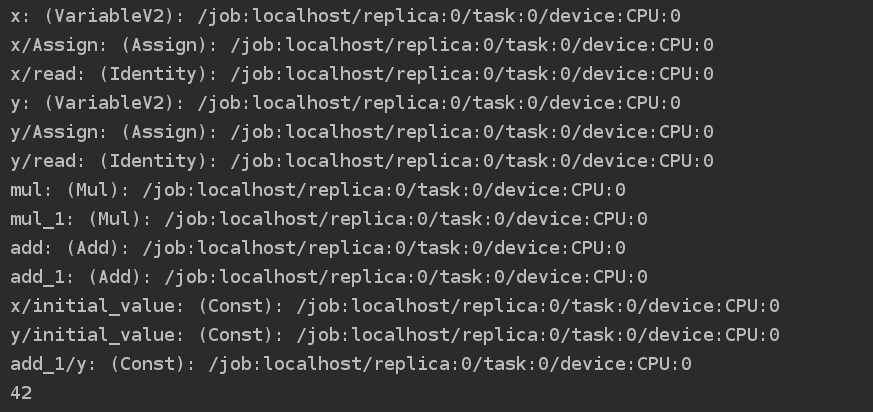## （3）图的管理

import  tensorflow as tf

x1 = tf.Variable(1)
print(x1.graph is tf.get_default_graph())

graph = tf.Graph()
x3 = tf.Variable(3)
with graph.as_default():
x2 = tf.Variable(2)

x4 = tf.Variable(4)
print(x2.graph is graph)
print(x2.graph is tf.get_default_graph())

print(x3.graph is tf.get_default_graph())
print(x3.graph is graph)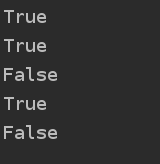## （4） lifecycle

import  tensorflow as tf

w = tf.constant(3)
x = w+2
y = x+5
z= x*3

with tf.Session() as sess:
print(y.eval())
print(z.eval())

print("*********************")
with tf.Session() as sess2:
y_val , z_val = sess2.run([y,z])
print(y_val)
print(z_val)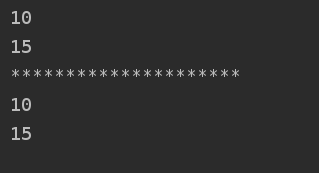## （5） autodiff

TensorFlow 提供的autodiff特性可以自动的并有效的为我们计算梯度

import tensorflow as tf
import numpy as np
from sklearn.datasets import  fetch_california_housing
from sklearn.preprocessing import  StandardScaler

n_epochs = 1000
learning_rate = 0.01

m ,n = housing.data.shape
housing_data_plus_bias = np.c_[np.ones((m,1)),housing.data]

scaler = StandardScaler().fit(housing_data_plus_bias)
scaled_housing_data_plus_bias = scaler.transform(housing_data_plus_bias)

X = tf.constant(scaled_housing_data_plus_bias,dtype = tf.float32,name = 'X')
y = tf.constant(housing.target.reshape(-1,1),dtype = tf.float32,name='y')

theta = tf.Variable(tf.random_uniform([n+1,1],-1.0,1.0),name = 'theta')
y_pre =  tf.matmul(X,theta,name= 'predictions')
error = y_pre - y
mse = tf.reduce_mean(tf.square(error),name = 'mse')

init = tf.global_variables_initializer()

with tf.Session() as sess:
sess.run(init)

for epoch in range(n_epochs):
if epoch %100 ==0 :
print("epoch",epoch,'MSE=', mse.eval())

sess.run(training_op)

best_theta = theta.eval()
print(best_theta)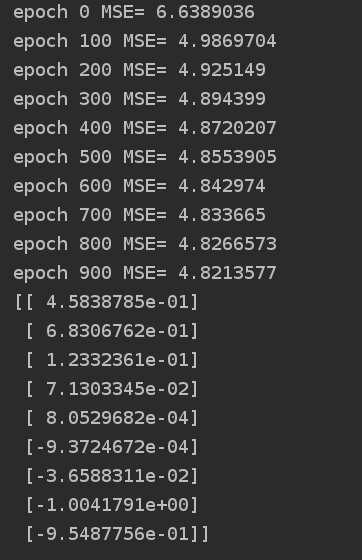## （6） placeholder

placeholder是一个占位符
TensorFlow中的占位符，用于传入外部数据。

• dtype:数据类型。
• shape:数据的维度。默认为None，表示没有限制
• name:名称

feed_dict : 是需要传入的值（是一个字典）

import tensorflow as tf

A = tf.placeholder(tf.float32, shape=(None, 3))
B = A + 5

with tf.Session() as  sess:
B_val_1 = B.eval(feed_dict={A: [[1, 2, 3]]})
B_val_2 = B.eval(feed_dict={A: [[4, 5, 6], [7, 8, 9]]})

print(B_val_1)
print(B_val_2)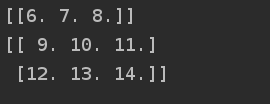©️2019 CSDN 皮肤主题: 大白 设计师: CSDN官方博客